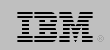# IBM "Classic" REXX

Implementation of programming language REXX

“Classic” REXX is the original implementation, created for IBM’s VM/SP CMS operating system, then ported to MVS, VSE, OS/2 and AIX. REXX is an acronym for REstructured eXtended eXecutor. REXX later attained an ANSI standard specification, and has been ported to many other platforms. The Classic implementation follows almost all of the ANSI standard. Though it supports stream I/O, usually an alternate, EXECIO, is used to communicate with the OS.IBM logo

## Examples:

### Hello, World!:

Example for versions REXX, Regina 3.3
``````say 'Hello, World!'
``````

### Factorial:

Example for versions REXX

This example uses recursive factorial definition.

Line 3: REXX allows the numeric precision to be set arbitrarily large — as much as your memory will allow.

Line 6: String concatenation works by juxtaposing values and literals, with or without intervening spaces. If two values must be concatenated, the || operator can be used.

Line 10: The optional PROCEDURE keyword causes the routine’s variables to be local; without it they are global to the program.

The output looks as follows:

0! = 1
1! = 1
2! = 2
3! = 6
4! = 24
5! = 120
6! = 720
7! = 5040
8! = 40320
9! = 362880
10! = 3628800
11! = 39916800
12! = 479001600
13! = 6227020800
14! = 87178291200
15! = 1307674368000
16! = 20922789888000
17! = 355687428096000
18! = 6402373705728000
19! = 121645100408832000
20! = 2432902008176640000
21! = 51090942171709440000
22! = 1124000727777607680000
23! = 25852016738884976640000
24! = 620448401733239439360000
25! = 15511210043330985984000000
26! = 403291461126605635584000000
27! = 10888869450418352160768000000
28! = 304888344611713860501504000000
29! = 8841761993739701954543616000000
30! = 265252859812191058636308480000000

``````  1  #!/usr/bin/rexx
2  /* Compute n! */
3    numeric digits 40
4
5    do n = 0 to 30
6      say right(n,2)"! = " right(factorial(n),35)
7    end
8  exit
9
10  factorial: procedure
11    parse arg n .
12
13    if n = 0 then
14      n = 1
15
16    else
17      n = n * factorial(n - 1)
18  return n
``````

### Fibonacci numbers:

Example for versions REXX

This example uses recursive definition of Fibonacci numbers.

`````` 1  #!/usr/bin/rexx
2  /* Calculate Fibonacci using recursion */
3
4    numbers = ''
5
6    do n = 1 to 16
7      numbers = numbers fibonacci(n)","
8    end
9
10    say numbers"..."
11  exit
12
13  fibonacci: procedure
14    parse arg n .
15
16    if n < 3 then
17      n = 1
18
19    else
20      n = fibonacci(n-1) + fibonacci(n-2)
21  return n
``````

### Fibonacci numbers:

Example for versions REXX

This example uses iterative definition of Fibonacci numbers.

In REXX, an uninitialized variable has its name in uppercase as its value; e.g. numbers = ‘NUMBERS’

Line 4: Arrays are called “stem variables”. The root of a stem ends with a period. Elements of a stem are named by adding a value to the stem; e.g. fib.1, fib.first, etc. Additional dimensions may be used by adding another period and value; e.g., fib.1.3; To initialize all possible stem instances, assign a value to the stem name; e.g., fib. = 0 or fib. = ”

`````` 1  #!/usr/bin/rexx
2  /* Calculate Fibonacci using an associative array */
3
4    fib.  = ''
5    fib.1 = 1
6    fib.2 = 1
7    numbers = ''
8
9    do f = 3 to 16
10      e = f - 1; d = f - 2
11      fib.f = fib.e + fib.d
12    end
13
14    do n = 1 to 16
15      numbers = numbers fib.n','
16      fib.f = fib.e + fib.d
17    end
18
19    say numbers"..."
20  exit
``````